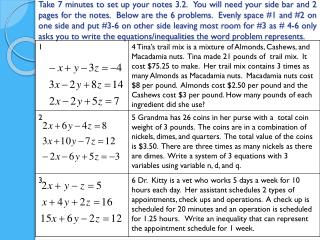DownloadDownload PresentationNotes Day 3.2 eq and inequalities

Notes Day 3.2 eq and inequalities

Download PresentationNotes Day 3.2 eq and inequalities

- - - - - - - - - - - - - - - - - - - - - - - - - - - E N D - - - - - - - - - - - - - - - - - - - - - - - - - - -
Presentation Transcript

1. Take 7 minutes to set up your notes 3.2. You will need your side bar and 2 pages for the notes. Below are the 6 problems. Evenly space #1 and #2 on one side and put #3-6 on other side leaving most room for #3 as # 4-6 only asks you to write the equations/inequalities the word problem represents.

2. Notes Day 3.2 eq and inequalities Solve systems in 3 variables Solve word problems in 3 var.

3. Summarize this later in the side bar after you have done a couple problems – I will put this slide in the presentation later for that purpose! • Process to solve systems in 3 variables (in general): • Pick 2 equations and make a variable add out using elimination • Pick 2 different equations and make the SAME variable add out • Now you have a system in 2 variables – like Algebra 1 (contin) • However, every problem is different: • Sometimes you can add equations right away and 2 var. may add out • Then you would use substitution in 2 equations to get those equations down to a 2 var. 2 equation Algebra 1 problem Use Elimination and Substitution multiple times to get 3 var eq. down so 2 of the equations have only 2 var and then solve Like you did in Algebra 1

4. Solve the system of 3 variables 1. Multiply by 2 X by 2 ADD! ADD!

5. Solve the system of 3 variables 2. Sub in 5 X by 3 You Try Now With a partner Add 1st and 3rd X by -2 ADD!

6. Now You Try 3. Hint: pick 2 eq. and make a var. add out using elim then pick another 2 eq. and use elim to make SAME var add out! + Multiply by 2 + Multiply by -3 Multiply by 5 +

7. 4. Tina’s trail mix is a mixture of Almonds, Cashews, and Macadamia nuts. Tina made 21 pounds of her trail mix to sell at the state fair and it cost \$75.25 to make. Her trail mix contains 3 times as many Almonds as Macadamia nuts. Macadamia nuts cost \$8 per pound. Almonds cost \$2.50 per pound and the Cashews cost \$3 per pound. How many pounds of each ingredient did she use? (I showed how to solve in case you want more examples at home on solving - just set up eq in class!) ADD

8. 5. Grandma has 26 coins in her purse with a total coin weight of 3 pounds. The coins are in a combination of nickels, dimes, and quarters. The total value of the coins is \$3.50. There are three times as many nickels as there are dimes. Write a system of 3 equations with 3 variables using variable n, d, and q. (I showed how to solve in case you want more examples at home on solving – just set up equations in class) ADD

9. 6. Dr. Kitty is a vet who works 5 days a week for 10 hours each day. Her assistant schedules 2 types of appointments, check ups and operations. A check up is scheduled for 20 minutes and an operation is scheduled for 1.25 hours. Write an inequality that can represent the appointment schedule for 1 week. Find hrs vs. min Not enough information to solve but write an inequality 2 ways:

10. Summarize this in the side bar • Process to solve systems in 3 variables (in general): • Pick 2 equations and make a variable add out using elimination • Pick 2 different equations and make the SAME variable add out • Now you have a system in 2 variables – like Algebra 1 (contin) • However, every problem is different: • Sometimes you can add equations right away and 2 var. may add out • Then you would use substitution in 2 equations to get those equations down to a 2 var. 2 equation Algebra 1 problem Use Elimination and Substitution multiple times to get 3 var eq. down so 2 of the equations have only 2 var and then solve Like you did in Algebra 1